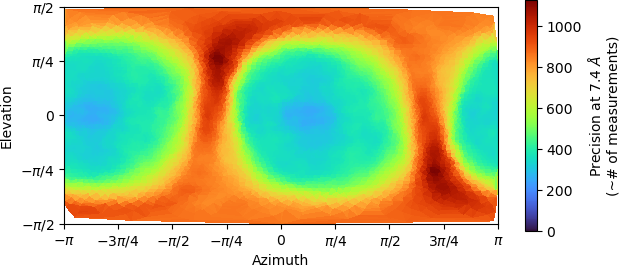# Posterior precision plot meaning

Hi!
I would like to know the meaning of Precision in this plot after refinements:Thanks!

The posterior precision plot is another way of looking at the diversity of views present in your dataset, complimentary to the viewing direction plot.

You can picture your structure at the center of a large circumscribed sphere. The elevation, azimuth angles define the direction of a radial line segment pointing from the center of the structure (i.e. the center of the sphere) out to the edge of the sphere. If you’re familiar with latitude and longitude: elevation is equivalent to latitude, and azimuth is equivalent to longitude. With this:

• The Viewing Direction Distribution histogram shows you how many images have a viewing direction at each (elevation, azimuth) bin.
• The Posterior Precision plot shows you roughly how many images contributed to the voxels that lie along this radial line segment.

It’s a bit harder to interpret than the viewing direction plot, but the posterior more accurately tells you how many observations contributed to the structure along every possible direction, and an ideal distribution might have values that all are within the same order of magnitude. In this case, the precision plot is fairly good – the most represented directions have a relative precision of ~1000, and the least represented have around ~300 or so, which are both within one order of magnitude difference.

Best,
Michael

3 Likes

Now I understand. Thank you so much!!

Thank you mmclean for the explanation. Is the histogram contribution weighted based on the likelihood probability for the different images?

Yes, each image’s contribution to the histogram’s values is proportional to the likelihood of its alignments, which comes directly from the residual between the raw data and the volume projections. The weights are also influenced by the CTF of each image (where the CTF of an image is zero, it doesn’t contribute to the structure, etc.).

Best,
Michael

1 Like